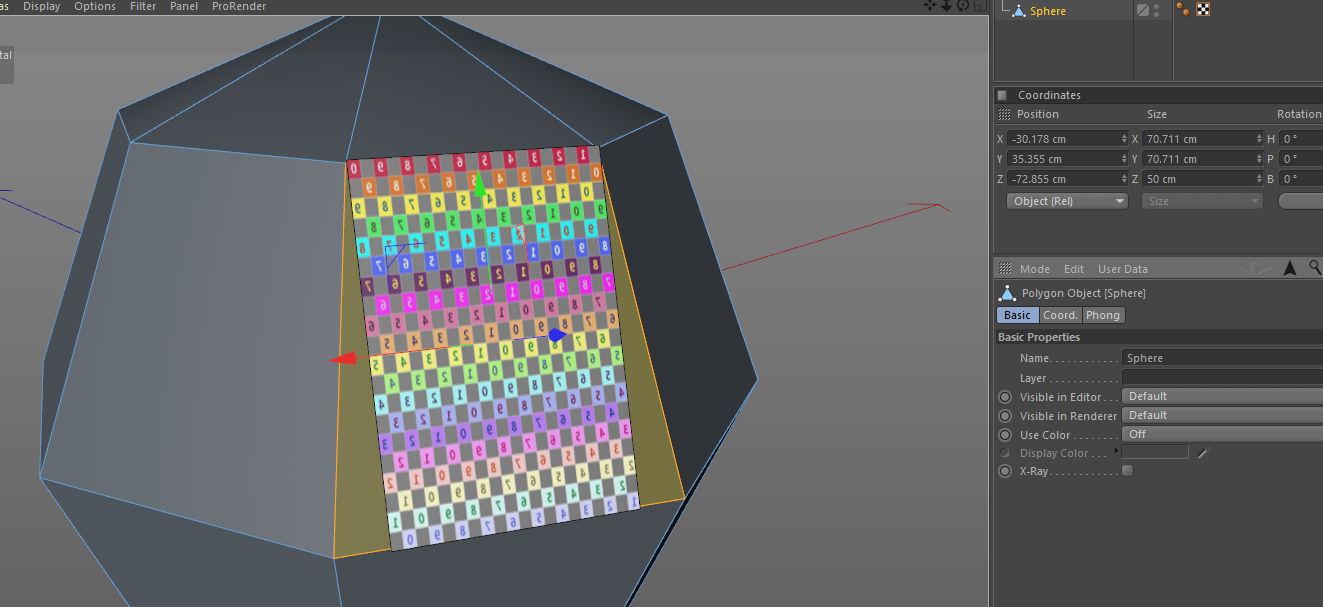# Polygon dimensions

• I want to fit images in selected polygons.
To do so, I get the polygon and then I like to get the smallest width and the smallest height of the polygon.
With this rectangle information, I create a plane and place one image on the plane.
The plane is then placed using the normal of the polygon.

Question: is there an easy way to get the smallest width and height of a polygon.

See for example this pictureHere how I calculate the width and height.

``````p = obj.GetPolygon(index)

a = allp[p.a]
b = allp[p.b]
c = allp[p.c]
d = allp[p.d]

w1 = (a - d).GetLength()
w2 = (b - c).GetLength()
h1 = (a - b).GetLength()
h2 = (d - c).GetLength()

width = w1
if (w2 < w1): width = w2
height = h1
if (h2 < h1): height = h2
``````

This code does not seem correct, because the Coordinate Manager show a different size.

Also, the sequence of the points (a,b,c,d) is not always the same.
Sometimes it starts in the lower left corner and sometime in the upper right corner.

• First, the coordinate manager is not showing the dimensions of the polygon. It's showing the dimensions of the enclosing axis-parallel cuboid, where the axes are either the axes of the object or the world system. So you will not get the width and height information from that (at least not in the way your image suggests).

The point order is arbitrary. I'm not sure why you expect the points to start in a certain corner?

Second, your code is extremely specific. A polygon may be nonplanar or rotated in space. The sides of a polygon may not be parallel (as in square, rectangle, parallelogram or trapeze), and even if some sides are parallel, they don't necessarily are parallel to any axis system (e.g. to the ground). I do not know how you place your plane and image, but it looks as if the code would only work if the two sides used as "width" are parallel to ground level (and even then the height is wrong for a trapeze as shown in the image).

To solve the projection, you need to define first what "width" and "height" should be for an arbitary polygon (a parallelogram e.g. has two heights...), and what kind of restrictions you make on the input (I assume you will only handle planar quads, but do you want to rotate the image, or should it always be parallel to the ground? Do you want to maximize image size on the polygon? Do you want the quads to be trapezes only? Do some quad edges need to be parallel to the ground or not?). Once you have that parameters, you can start thinking about how these widths and heights can be calculated.

(For measures of classic polygons, you can always google "height parallelogram" e.g.)

• Thanks for the detailed answer.
And yes, I guess my approach is way to simple.
However, I use the normal of the polygon to place the plane and getting the minimal width and height, best fits a planar polygon.

"The point order is arbitrary. I'm not sure why you expect the points to start in a certain corner?"
For consistency I would expect the points to be in a predefined sequence.
Now it seems randomly (clockwise, anti clockwise, were is point a located?).

• Hi Pim, thanks for reaching out us.

With regard to your question, given for comments from Cairyn, I recommend to refine your approach and to look on the web since there are a few different strategies to find the largest inscribed rectangle in a non-convex polygon (among them I recommend having a look at this).

With regard to the points walking direction for a given polygon this is defined by the polygon normal and the left-hand rule and it should definitively not be randomly defined.

Cheers, Riccardo